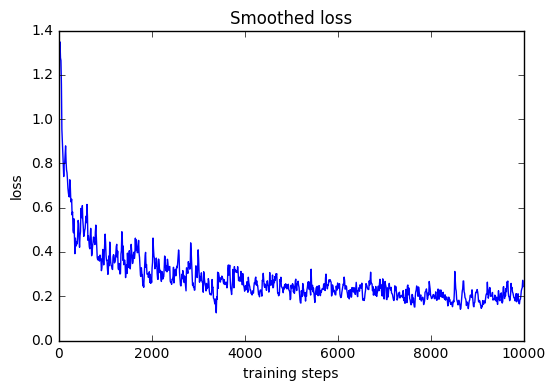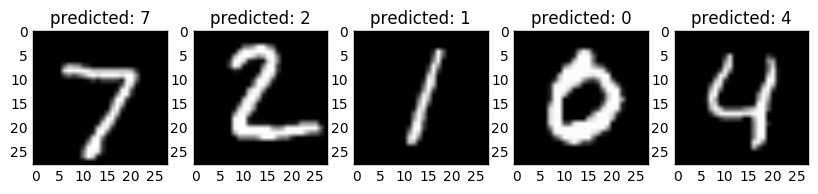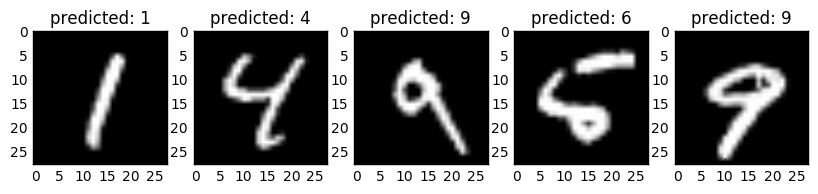# MNIST neural network demo¶

### A project by Sam Greydanus. MIT license¶

This is a vanilla two-layer neural network for solving the MNIST written digit classification task. When I was first learning how to use neural networks, backpropagation was by far the most confusing part.

I've broken the gradient computations into several smaller functions and included mathematical explanations of each step to make backprop more transparent

In :
import numpy as np
import matplotlib.pyplot as plt
import matplotlib.cm as cm
%matplotlib inline

from tensorflow.examples.tutorials.mnist import input_data

Extracting MNIST_data/train-images-idx3-ubyte.gz
Extracting MNIST_data/train-labels-idx1-ubyte.gz
Extracting MNIST_data/t10k-images-idx3-ubyte.gz
Extracting MNIST_data/t10k-labels-idx1-ubyte.gz


## Hyperparameters¶

In :
resume = False
batch_size = 16
lr = 2e-2
reg = 1e-4
h1_size = 100 # first hidden layer
h2_size = 10 # second hidden layer
D = 28*28 # dimensionality


## Model Initialization¶

In :
model = {}
# first layer
model['W1'] = np.random.randn(D,h1_size) / np.sqrt(h1_size) # Xavier initialization
model['b1'] = np.random.randn(1,h1_size) / np.sqrt(h1_size) # see http://jmlr.org/proceedings/papers/v9/glorot10a/glorot10a.pdf
#second layer
model['W2'] = np.random.randn(h1_size,h2_size) / np.sqrt(h2_size)
model['b2'] = np.random.randn(1,h2_size) / np.sqrt(h2_size)


## Forward propagation¶

### x $\cdot$ W+b function¶

The x $\cdot$ W+b operation is just a linear transformation plus a constant. For a given input $x_j$ and output $h_k$ we have: $$h_k = x_jW_{jk} + b_k$$

In :
def xW_plus_b(x, W, b):
return np.dot(x,W) + b # in some cases you can even drop the bias b


### Softmax function¶

The softmax function is $p_k=\frac{e^{z_k}}{\sum_i e^{z_i}}$

In :
def softmax(x):
maxes = np.amax(x, axis=1, keepdims=True)
e = np.exp(x - maxes) # improves numerics
dist = e / np.sum(e, axis=1, keepdims=True)
return dist


### ReLU function¶

The ReLU function is $z_i=relu(h_i)$ where

$$relu(h_i) = \left\{ \begin{array}{ll} h_i & \quad h \ge 0\\ 0 & \quad \mathrm{otherwise} \end{array} \right.$$
In :
def relu(x):
x[x<0] = 0
return x


### Putting it together¶

In :
def forward(X, model):
# evaluate class scores, [N x K]
hs = [] # we'll need the h's for computing gradients

z1 = xW_plus_b(X, model['W1'], model['b1'])
h1 = relu(z1); hs.append(h1)

z2 = xW_plus_b(h1, model['W2'], model['b2'])
h2 = relu(z2); hs.append(h2)

probs = softmax(h2)
return probs, hs


## Backpropagation¶

The original softmax function is: $$p_k=\frac{e^{z_k}}{\sum_i e^{z_i}}$$ We're using a cross entropy loss function which looks like $$L_i= \left\{ \begin{array}{ll} y - log(p_k) & \quad y = k \\ -log(p_k) & \quad \mathrm{otherwise} \end{array} \right.$$ Taking the gradient of $L$ with respect to $f_k$, we get $$\frac{\partial L_i}{\partial z_k}=\frac{\partial}{\partial z_k}\left(-log(e^{z_k}) + log(\sum_i e^{z_i}) \right) = \frac{\partial}{\partial z_k}\left(-z_k + log(e^{z_1} + \ldots + e^{z_k} + \ldots + e^{z_n}) \right)$$ $$\frac{\partial L_i}{\partial z_k}=-\mathbb{1}(y_i=k) + \frac{e^{z_k}}{(e^{z_1} + \ldots + e^{z_k} + \ldots + e^{z_n})}$$ $$\frac{\partial L_i}{\partial z_k}= -\mathbb{1}(y_i=k) + p_k$$ Now we know how to calculate the whole gradient $\frac{\partial L}{\partial z_k}$

In :
def dsoftmax(h, y, batch_size):
h[range(batch_size),y] -= 1
return h/y.shape # divide by batch size


Propagating the gradient through the ReLU looks like: $$\frac{\partial L}{\partial z_k} \frac{\partial z_k}{\partial h_k} =\frac{\partial}{\partial z_k}\left( relu(h_k) \right) = \left\{ \begin{array}{ll} \frac{\partial L}{\partial z_k} * 1 & \quad h \ge 0\\ 0 & \quad \mathrm{otherwise} \end{array} \right.$$ Since we know $\frac{\partial L}{\partial z_k}$ from calculations above, we can also calculate $\frac{\partial L}{\partial h_k}$

In :
def drelu(dz, h):
dz[h <= 0] = 0 # backprop relu
return dz


### Gradient of x $\cdot$ W+b¶

The x $\cdot$ W+b operation is just a matrix dot product plus a constant vector $b$. We can break it into indices as follows: $$h_k = x_j*W_{jk} + b_k$$

Now we just need to propagate the gradient through this function.

$$\frac{\partial L}{\partial h_k} \frac{\partial h_k}{\partial x_j} = \frac{\partial L}{\partial h_k} \frac{\partial}{\partial x_j} \left( x_j*W_{jk} + b_k \right) = \frac{\partial L}{\partial h_k} W_{jk}$$

In practice, we'd like to vectorize this calculation. The best way to do this is with a matrix dot product $$\frac{\partial L}{\partial x_j} = \frac{\partial L}{\partial h} \cdot W^\intercal$$

In :
def dxW_plus_b(dh, model):
return np.dot(dh, model['W2'].T)


### Putting it together¶

In :
def backward(y, probs, hs, model):
grads = { k : np.zeros_like(v) for k,v in model.items() }
dh2 = dsoftmax(probs, y, batch_size)

# second hidden layer
print(hs.T.shape, dh2.shape, model['W2'].shape)

# first hidden layer
dh1 = dxW_plus_b(dh2, model)
dh1 = drelu(dh1, hs) # backprop through relu


## Evaluation metric¶

In :
# evaluate training set accuracy
def eval_model(model):
X = mnist.test.images
y = mnist.test.labels
hidden_layer = np.maximum(0, np.dot(X, model['W1']) + model['b1'])
scores = np.dot(hidden_layer, model['W2']) + model['b2']
predicted_class = np.argmax(scores, axis=1)
return (np.mean(predicted_class == y))


### A note on regularization¶

I've added L2 regularization to the cost and gradients here even though I didn't touch on it in the Gradients section above. Here's how it works.

Overfitting is a problem which occurs when a model fits itself to individual examples in training data. This prevents it from generalizing well on new data. One way that neural networks overfit is by making certain weights very large; we use L2 Regularization to prevent this.

The idea of L2 regularization is to add a penalty - the regularization loss - to the loss function which scales with the sum square of all parameters in the model. $$L_{\mathrm{regularization}} = \frac{1}{2} \gamma \theta \cdot \theta$$

Where $L_\mathrm{regularization}$ is regularization loss, $\gamma$ is a scaling hyperparameter, and $\theta$ is an 1D vector of all the training parameters. The gradient, then, has the simple form of

$$\frac{\partial L_\mathrm{regularization}}{\partial \theta} = \gamma \theta$$
In :
loss_history = []
smoothing_factor = 0.95
running_loss = 0
for i in range(2):
X, y = mnist.train.next_batch(batch_size)

probs, hs = forward(X, model)

# compute the loss: average cross-entropy loss and L2 regularization
y_logprobs = -np.log(probs[range(batch_size),y]) # we want probs on the y labels to be large
reg_loss = 0.5*reg*np.sum([np.sum(w*w) for w in model.values()])
loss = np.sum(y_logprobs)/batch_size + reg_loss

# update parameters

# boring book-keeping
running_loss = smoothing_factor*running_loss + (1-smoothing_factor)*loss
if i % 1000 == 0: print("iteration {}: test accuracy {:3f}".format(i, eval_model(model)))
if i % 250 == 0: print("\titeration %d: loss %f" % (i, running_loss))
if i % 10 == 0: loss_history.append((i,running_loss))

(100, 16) (16, 10) (100, 10)
iteration 0: test accuracy 0.925300
iteration 0: loss 0.012139
(100, 16) (16, 10) (100, 10)


## Visualize training loss¶

In :
plt.title("Smoothed loss")
plt.xlabel("training steps")
plt.ylabel("loss")

train_steps, smoothed_losses = zip(*loss_history)
plt.plot(train_steps, smoothed_losses)
plt.show()## Sample model¶

In :
X = mnist.test.images
y = mnist.test.labels
hidden_layer = np.maximum(0, np.dot(X, model['W1']) + model['b1'])
scores = np.dot(hidden_layer, model['W2']) + model['b2']
predicted_class = np.argmax(scores, axis=1)

for i in range(0,10,5):
img1 = np.reshape(X[i+0,:], (28,28))
img2 = np.reshape(X[i+1,:], (28,28))
img3 = np.reshape(X[i+2,:], (28,28))
img4 = np.reshape(X[i+3,:], (28,28))
img5 = np.reshape(X[i+4,:], (28,28))
plt.figure(i, figsize=(10,4))
plt.subplot(151) ; plt.title("predicted: " + str(predicted_class[i]))
plt.imshow(img1, cmap=cm.gray)
plt.subplot(152) ; plt.title("predicted: " + str(predicted_class[i+1]))
plt.imshow(img2, cmap=cm.gray)
plt.subplot(153) ; plt.title("predicted: " + str(predicted_class[i+2]))
plt.imshow(img3, cmap=cm.gray)
plt.subplot(154) ; plt.title("predicted: " + str(predicted_class[i+3]))
plt.imshow(img4, cmap=cm.gray)
plt.subplot(155) ; plt.title("predicted: " + str(predicted_class[i+4]))
plt.imshow(img5, cmap=cm.gray)